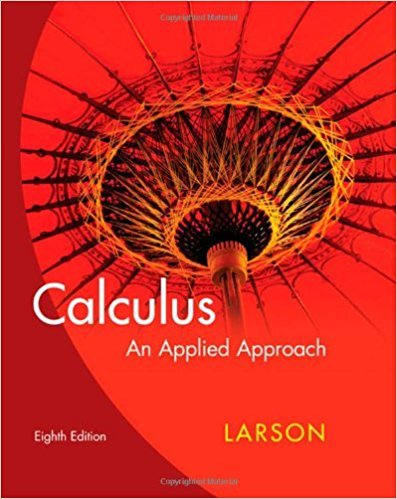×
×

# Solutions for Chapter 7: Functions of Several Variables## Full solutions for Calculus: An Applied Approach | 8th Edition

ISBN: 9780618958252Solutions for Chapter 7: Functions of Several Variables

Solutions for Chapter 7
4 5 0 407 Reviews
30
5
##### ISBN: 9780618958252

Since 100 problems in chapter 7: Functions of Several Variables have been answered, more than 23649 students have viewed full step-by-step solutions from this chapter. This expansive textbook survival guide covers the following chapters and their solutions. Chapter 7: Functions of Several Variables includes 100 full step-by-step solutions. Calculus: An Applied Approach was written by and is associated to the ISBN: 9780618958252. This textbook survival guide was created for the textbook: Calculus: An Applied Approach , edition: 8.

Key Calculus Terms and definitions covered in this textbook
• Bearing

Measure of the clockwise angle that the line of travel makes with due north

• Confounding variable

A third variable that affects either of two variables being studied, making inferences about causation unreliable

• Constant

A letter or symbol that stands for a specific number,

• Division

a b = aa 1 b b, b Z 0

• Graphical model

A visible representation of a numerical or algebraic model.

• Infinite discontinuity at x = a

limx:a + x a ƒ(x) = q6 or limx:a - ƒ(x) = q.

• Law of sines

sin A a = sin B b = sin C c

• Natural logarithm

A logarithm with base e.

• NINT (ƒ(x), x, a, b)

A calculator approximation to ?ab ƒ(x)dx

• Periodic function

A function ƒ for which there is a positive number c such that for every value t in the domain of ƒ. The smallest such number c is the period of the function.

• Polynomial function

A function in which ƒ(x)is a polynomial in x, p. 158.

• Quotient polynomial

See Division algorithm for polynomials.

• Random numbers

Numbers that can be used by researchers to simulate randomness in scientific studies (they are usually obtained from lengthy tables of decimal digits that have been generated by verifiably random natural phenomena).

• Rational expression

An expression that can be written as a ratio of two polynomials.

• Resistant measure

A statistical measure that does not change much in response to outliers.

• Right triangle

A triangle with a 90° angle.

• Simple harmonic motion

Motion described by d = a sin wt or d = a cos wt

A graph in which (-x, y) is on the graph whenever (x, y) is; or a graph in which (-r, -?) or (r, ?, -?) is on the graph whenever (r, ?) is

• Symmetric matrix

A matrix A = [aij] with the property aij = aji for all i and j

• Vertical component

See Component form of a vector.

×